## Chapter Goals¶

1. Understand the basics of stationarity
2. Understand how asset returns violate the rules of stationarity
3. Demonstrate that concept that asset returns can be considered as being generated by different distributions
4. Motivating the use of a more robust prediction methodology    

## Chapter Outline¶

1. Getting and Storing a Dataset
2. Defining Stationarity
3. How Asset Returns Violate Rules of Stationarity
4. Conclusions

## 1. Getting and Storing a Dataset¶

Start by importing the required modules and tools

In :
%load_ext watermark
%watermark

from IPython.display import display

# import standard libs
from pathlib import PurePath, Path
import sys
import time

# get project dir
pp = PurePath(Path.cwd()).parts[:-1]
pdir = PurePath(*pp)
script_dir = pdir / 'scripts'
sys.path.append(script_dir.as_posix())

# import python scientific stack

import pandas as pd
pd.options.display.float_format = '{:,.4f}'.format
import numpy as np
import sklearn.mixture as mix
import statsmodels.tsa.api as smt
import statsmodels.api as sm
import scipy.stats as stats

# import visual tools

from mpl_toolkits import mplot3d
import matplotlib as mpl
import matplotlib.pyplot as plt
import matplotlib.gridspec as gridspec
%matplotlib inline
import plotnine as pn
import mizani.breaks as mzb
import mizani.formatters as mzf
import seaborn as sns

# import util libs
import pyarrow as pa
import pyarrow.parquet as pq
import warnings
warnings.filterwarnings("ignore")
from utils import cprint

# set globals
plt.style.use('seaborn-ticks')
plt.rcParams['font.family'] = 'DejaVu Sans Mono'
plt.rcParams['font.size'] = 9.5
plt.rcParams['font.weight'] = 'medium'
plt.rcParams['figure.figsize'] = 10,7

blue, green, red, purple, gold, teal = sns.color_palette('colorblind', 6)
RANDOM_STATE = 777

print()

The watermark extension is already loaded. To reload it, use:
2017-12-12T11:47:44-07:00

CPython 3.6.2
IPython 6.1.0

compiler   : GCC 4.8.2 20140120 (Red Hat 4.8.2-15)
system     : Linux
release    : 4.10.0-38-generic
machine    : x86_64
processor  : x86_64
CPU cores  : 12
interpreter: 64bit

pandas 0.21.0
numpy 1.13.1
sklearn 0.19.0
statsmodels 0.8.0
scipy 1.0.0
matplotlib 2.1.0
seaborn 0.8.1
plotnine 0.3.0+17.gcde6d84
pyarrow 0.7.1


#### First let's get some market data from Yahoo. We'll get the adjusted closing price for multiple ETFs.¶

In :
get_price = lambda sym, start, end: web.DataReader(sym, 'yahoo', start, end)['Adj Close']

chosen_syms = ['SPY', 'QQQ', 'TLT', 'GLD', 'EFA', 'EEM']

end = pd.to_datetime('2017-09-30')
start = end - 25 * 252 * pd.tseries.offsets.BDay()

#%time df = (pd.DataFrame({sym:get_price(sym, start, end) for sym in chosen_syms}).dropna())
#cprint(df)


#### Next we create the return dataframe and save it using pyarrow.¶

Please note that although this dataset is small enough to save as csv or h5, if you intend on dealing with larger datasets aka "big data" on "distributed systems" I recommend developing the pyarrow habit now. This will save you time and storage costs down the road.

In :
# compute returns

#rs = np.log(df/df.shift(1)).dropna()
#cprint(rs)

In :
# simple save as parquet func
save_parquet = lambda df, save_fp: pq.write_table(pa.Table.from_pandas(df), save_fp)

#save_fp = pdir.as_posix() + f'/data/etf_returns_{rs.index.min().date()}-{rs.index.max().date()}.parquet'
#save_parquet(rs, save_fp)


After saving the data we can load back into the environment like so...

In :
# simple load func

.to_pandas() # convert to pandas df
.assign(year=lambda df: df.index.year)) # add year column for later conv.
cprint(rs)

-------------------------------------------------------------------------------
dataframe information
-------------------------------------------------------------------------------
EEM     EFA     GLD     QQQ     SPY     TLT  year
Date
2017-09-25 -0.0171 -0.0057  0.0104 -0.0106 -0.0020  0.0062  2017
2017-09-26 -0.0027 -0.0022 -0.0112  0.0026  0.0006 -0.0018  2017
2017-09-27 -0.0036  0.0007 -0.0095  0.0090  0.0039 -0.0152  2017
2017-09-28 -0.0007  0.0026  0.0019 -0.0003  0.0012 -0.0030  2017
2017-09-29  0.0114  0.0059 -0.0052  0.0072  0.0035  0.0022  2017
--------------------------------------------------
<class 'pandas.core.frame.DataFrame'>
DatetimeIndex: 3238 entries, 2004-11-19 to 2017-09-29
Data columns (total 7 columns):
EEM     3238 non-null float64
EFA     3238 non-null float64
GLD     3238 non-null float64
QQQ     3238 non-null float64
SPY     3238 non-null float64
TLT     3238 non-null float64
year    3238 non-null int64
dtypes: float64(6), int64(1)
memory usage: 202.4 KB
None
-------------------------------------------------------------------------------



Please be aware that the load function used above immediately converts the parquet file into a pandas.DataFrame object. For smaller datasets and modern laptop hardware that is not a problem (less than 1 gb). However once your dataset starts getting into the multi gb range one might experience delayed performance in which case I would recommend learning and using Dask.

## 2. Defining Stationarity¶

The following image sums up this concept best. The simple intuition is that the series mean and variance should NOT change as a result of passing time. The covariance between returns in the time series should also NOT be a function of time.

In :
from IPython.display import Image

media = './visuals/01_Motivation/stationarity infographic screenshot-www.seanabu.com-2016-11-01-14-16-35.png'
Image(media)

Out: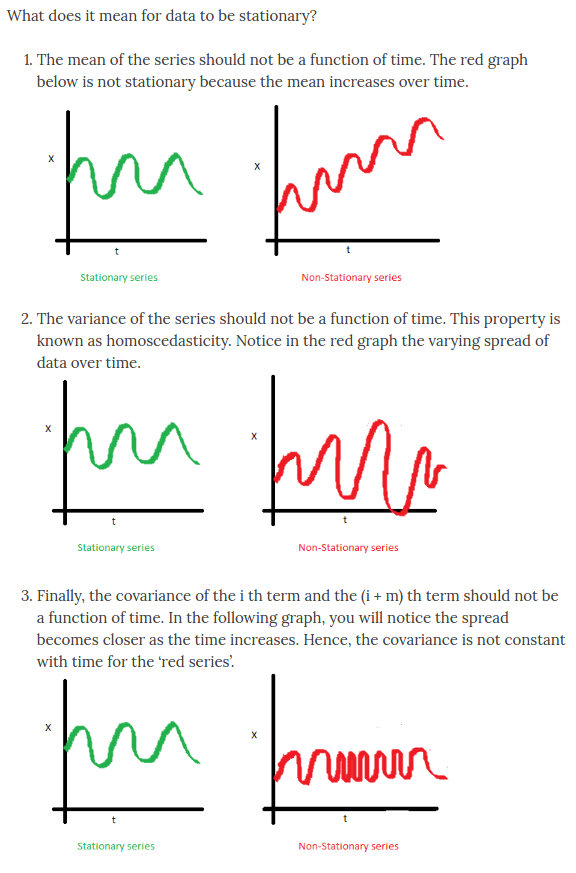## 3. How Asset Returns Violate Rules of Stationarity¶

Now that you are familiarized with the concept of stationary time series, the remainder of this notebook will demonstrate/discuss some of the nuances and challenges one runs into in when trying to predict time series.

Before we start just know that the behavior of securities' returns challenges these requirements of stationarity and our attempts to predict them in ways that are sometimes unknowable. This is a very humbling realization but is a necessary fact that requires a strategic response in the practice of return prediction.

The remainder of this notebook will use the canonical SPY etf as the primary example but I urge you to download the notebook and experiment with the other securities.

Below I define some convenience functions for plotting.

In :
def add_mean_std_text(x, **kwargs):
"""fn: add mean, std text to seaborn plot

# Args
x : pd.Series()
"""
mean, std = x.mean(), x.std()
mean_tx = f"mean: {mean:.4%}\nstd: {std:.4%}"

txkw = dict(size=14, fontweight='demi', color='red', rotation=0)
ymin, ymax = plt.gca().get_ylim()
plt.text(mean+0.025, 0.8*ymax, mean_tx, **txkw)
return

def plot_dist(rs, ex):
"""fn: to plot single distro with fitted histograms using FacetGrid

# Args
rs : pd.DataFrame(), return df
ex : str(), security/column name
"""
plt.style.use('dark_background')
plt.rcParams['font.size'] = 14
g = (rs
.pipe(sns.FacetGrid,
size=5,
aspect=1.5)
.map(sns.distplot, ex, kde=False, fit=stats.norm,
fit_kws={'color':green, 'lw':2.5, 'label':'norm'})
.map(sns.distplot, ex, kde=False, fit=stats.laplace,
fit_kws={'linestyle':'--', 'color':gold, 'lw':2.5, 'label':'laplace'})
.map(sns.distplot, ex, kde=False, fit=stats.johnsonsu,
fit_kws={'linestyle':'-', 'color':red, 'lw':2.5, 'label':'jsu'})
sns.despine(offset=1)
plt.title(f'{ex} returns')
return

def plot_facet_hist(rs, ex):
"""fn: to plot multiple fitted histograms using FacetGrid

# Args
rs : pd.DataFrame(), return df
ex : str(), security/column name
"""
plt.style.use('dark_background')

plt.rcParams['font.size'] = 12
df = rs.assign(year=lambda df: df.index.year)
g = (sns.FacetGrid(df, col='year',col_wrap=2, size=4, aspect=1.2) # make sure to add legend
.map(sns.distplot, ex, kde=False, fit=stats.norm,
fit_kws={'color':green, 'lw':2.5, 'label':'norm'})
.map(sns.distplot, ex, kde=False, fit=stats.laplace,
fit_kws={'linestyle':'--', 'color':gold, 'lw':2.5, 'label':'laplace'})
.map(sns.distplot, ex, kde=False, fit=stats.johnsonsu,
fit_kws={'linestyle':'-', 'color':red, 'lw':2.5, 'label':'jsu'})

sns.despine(offset=1)
return


#### First let's address the concept of normality. Are SPY returns normally distributed? (short answer: sometimes)¶

Let's look at the entire return distribution of SPY as a histogram using seaborn's distplot. You'll notice that I have layered fitted density plots using the following distributions: normal, laplace, and johnsonsu.

In :
ex = 'SPY'
df = rs.loc['2005':].copy() # use 2005 cutoff b/c it's first full year of data

plot_dist(df, ex)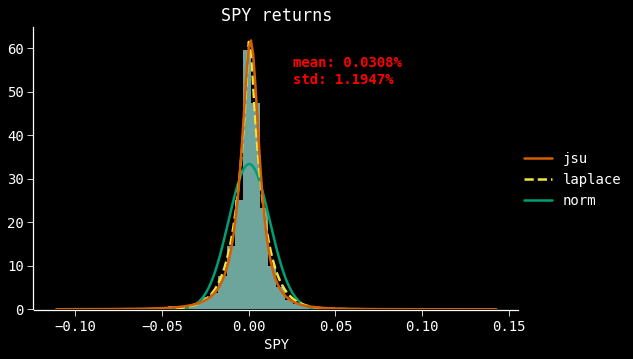Looking at the chart above we can see how badly the normal distribution approximates the dataset. Both laplace and jsu distributions do a much better job approximating the returns. All this proves is that the return distribution for the SPY etf, over the selected time period, is certainly not normal.

Let's explore this a bit further. We can use a quantile plot which which allows us to compare the quantile distribution of our parameter e.g. SPY returns, with that of a theoretical distribution, e.g. Normal.

In :
def quantile_plot(x, **kwargs):
"""fn: plot a quantile plot of returns vs normal distribution

# Args
x : pd.Series()
"""

plt.rcParams['font.family'] = 'dejavu sans mono'
res = stats.probplot(x, fit=True, plot=plt)
_slope, _int, _r = res[-1]

ax = plt.gca()
ax.get_lines().set_marker('s')
ax.get_lines().set_markerfacecolor('r')
ax.get_lines().set_markersize(13.0)
ax.get_children()[-2].set_fontsize(22.)

txkw = dict(size=14, fontweight='demi', color='r')
r2_tx = "r^2 = {:.2%}\nslope = {:.4f}".format(_r, _slope)

ymin, ymax = ax.get_ylim()
xmin, xmax = ax.get_xlim()
ax.text(0.5*xmax, .8*ymin, r2_tx, **txkw)
return

In :
s = df[ex]
quantile_plot(s)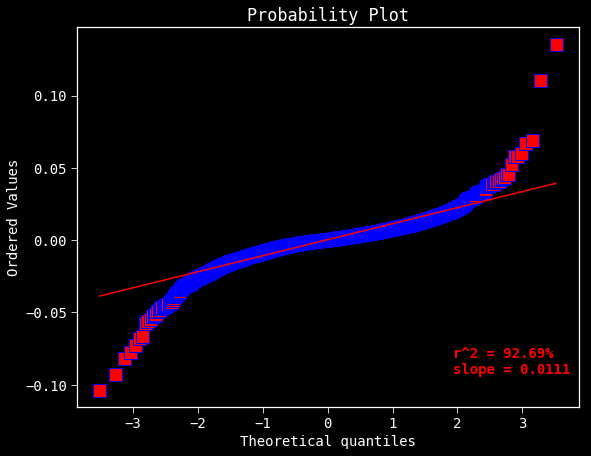As most of you already know SPY returns exhibit a phenomenon known as "fat tails" as clearly demonstrated by the above plot. All this means is that extreme returns, both positive and negative, occur at a much higher frequency than predicted by the normal distribution. When you read various papers, authors may also refer to this concept by saying "the distribution has more MASS in the TAILS". In this context they mean the same thing. So this shows that over the period of 12 years that SPY returns are not distributed according to the Normal distribution.

But, we haven't really addressed the stationary aspect, yet.

One way we can examine whether the returns are stationary is looking at the mean and standard deviation of returns by year. If those two quantities are not supposed to be a function of time then they should be pretty similar across years, right?

In :
%%time

plot_facet_hist(df, ex)
plt.savefig(f'./visuals/01_Motivation/spy return faceted histogram plot-mean std.png', dpi=300, bbox_inches='tight')

CPU times: user 14.9 s, sys: 6.71 s, total: 21.6 s
Wall time: 13.7 s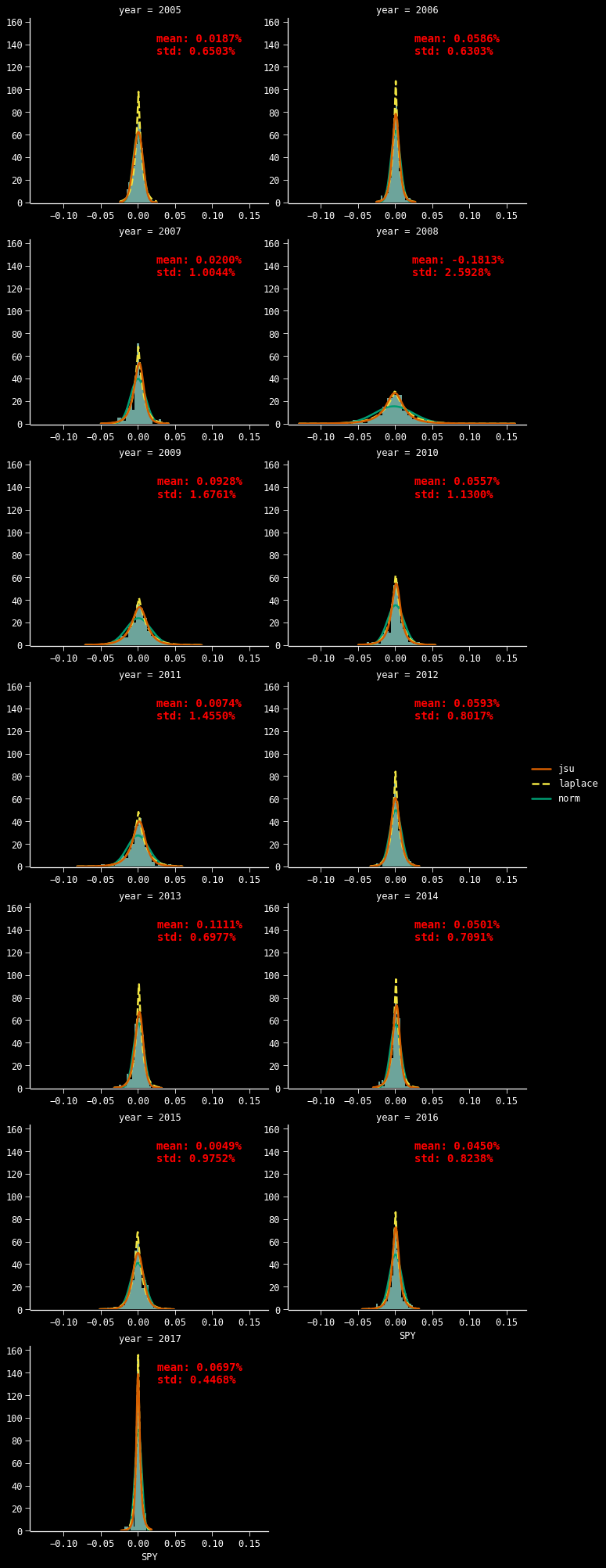#### The annual means and standard deviations vary signficantly across years.¶

Just by eyeballing the plots you can see how varied the return distribution is. Sometimes the distribution is highly peaked like 2006 and 2017, and other times it's wider and flatter, like 2008-09. We can see a little bit of that variation more clearly below.

In :
df.groupby('year')[ex].agg(['mean', 'std']).plot(marker='o', subplots=True);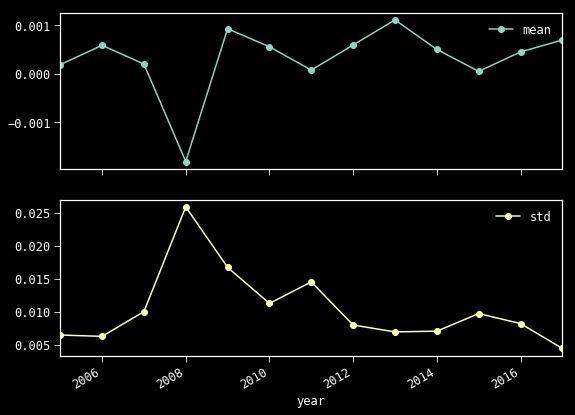Did you notice the fitted normal density plots in the faceted histogram looked like it did a better job of approximating the returns for the individual years? Does that mean that our previous conclusion that returns are NOT normally distributed is simply a matter of the time scale? Let's look into this a little further with a faceted quantile plot.

In :
def plot_facet_qq(rs, ex):
"""fn: to plot faceted quantile plots using FacetGrid

# Args
rs : pd.DataFrame()
ex : str(), security/column name
"""
g = (rs
.pipe(sns.FacetGrid,
col='year',
col_wrap=2,
size=7,
aspect=1.3)
.map(quantile_plot, ex)
sns.despine(offset=1, trim=True)
return

In :
%time plot_facet_qq(df, ex)
plt.savefig(f'./visuals/01_Motivation/spy return faceted quantile plot-kstest.png', dpi=300, bbox_inches='tight')

CPU times: user 1.66 s, sys: 952 ms, total: 2.61 s
Wall time: 1.47 s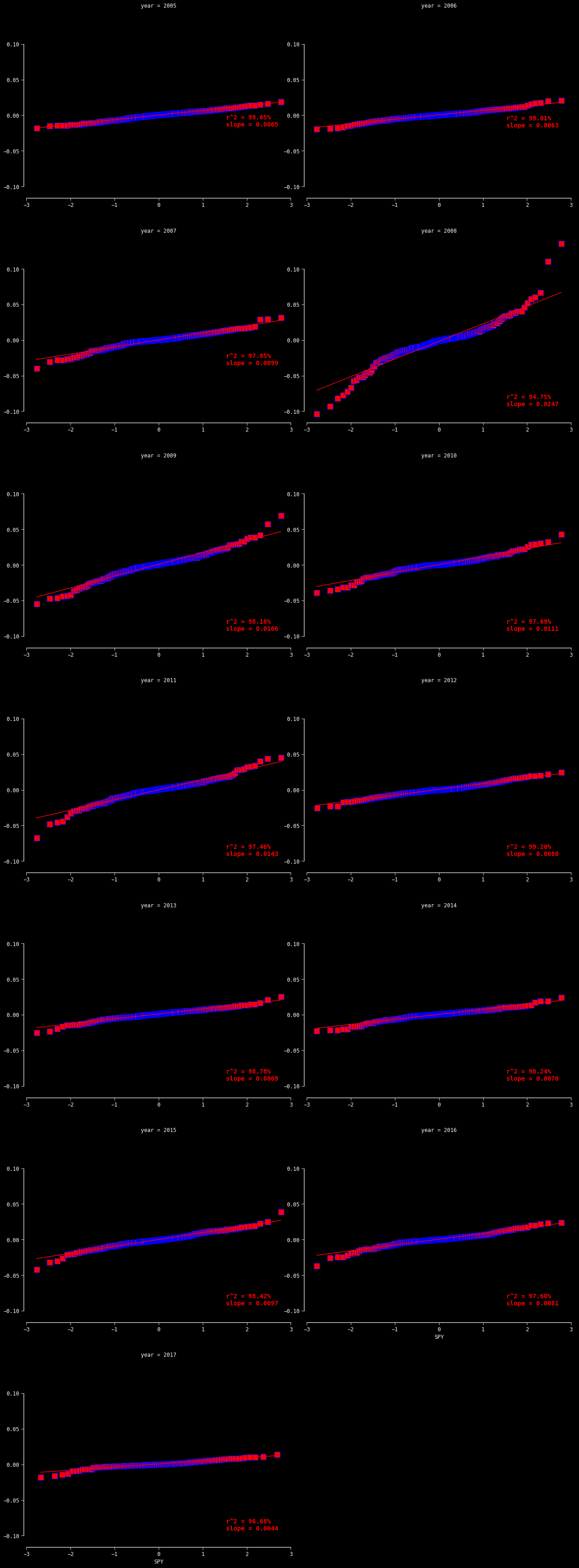Unsurprisingly we see that in certain years the return distribution is indistiguishable from a normally distributed approximation. In other years we see that the assumption of normality would have been disastrous. So we can say, by visual observation, that the means and variances of the return series are at minimum a function of time, a function of the time scale, and a function of the quantity of data points.

What we can also observe is the effect of central bank intervention on US listed multinational corporations and their asset prices. In 2005-06 we can see return distributions are effectively normally distributed. By 2007 we can see that some returns were more negative and occuring with more frequency than predicted using normal approximation. By 2008 the wheels were falling off the bus and the volatility and magnitude of the returns, both postive and negative, were highly non-normal. 2009-10 marks the time that the Fed stepped in to stabilize markets. However it was not enough, as the financial "contagion" had spread globally and especially to European markets. 2011 was the Euro-Crisis and we can see similar non-normal return pattern returns, especially negative returns. Then the global recapitalization aka global debt monetization scheme kicked in around 2012 and we've seen an unprecedented decline in volatility and return dispersion.

In :
norm = stats.norm

def generate_norm_rvs(ser, N=None):
if not N: N = ser.shape
return norm.rvs(ser.mean(), ser.std(), size=N, random_state=RANDOM_STATE)

def generate_norm_pdf(ser, N=None):
if not N: N = ser.shape
_min, _max = ser.min(), ser.max()
x = np.linspace(_min, _max, N)

y = norm.pdf(x, ser.mean(), ser.std())
return x, y

def generate_norm_cdf(ser, N=None):
if not N: N = ser.shape
_min, _max = ser.min(), ser.max()
x = np.linspace(_min, _max, N)

y = norm.cdf(x, ser.mean(), ser.std())
return x, y


#### The final test - Kolmogorov-Smirnov.¶

Let's test this more rigorously. For this we will use the CDF of the returns and compare those with a fitted or empirical CDF using the kolmogorov-smirnov two sample test (scipy.stats.kstest). This test will allow us to moore definitively state whether the CDF of the two series come from the same distribution e.g. the Normal distribution. the kstest outputs the ks statistic and a p-value. P-values less than 0.05 means we can reject the null hypothesis that the two series were drawn from the same distribution. The smaller the p-value the more confident we are that they came from different distributions.

First we will analyze the entire return series, then compare the test across years.

In :
def plot_cdf(ser, **kwds):
"""fn: to plot single CDF comparison

# Args
ser : pd.Series()
"""
plt.style.use('dark_background')
plt.rcParams['font.family'] = 'dejavu sans mono'

# plot kde
g = sns.kdeplot(ser, cumulative=True, lw=3, color=blue)

x, y = generate_norm_cdf(ser) # generate fitted normal CDF
g.plot(x, y, color=red, lw=3, label='fitted normal CDF')

# 2 sided ks test
#  https://docs.scipy.org/doc/scipy/reference/generated/scipy.stats.kstest.html
ks, p = stats.kstest(ser, 'norm', args=(ser.mean(), ser.std()))

xmin,xmax=plt.gca().get_xlim()
ymin,ymax=plt.gca().get_ylim()

txkw = dict(size=14, fontweight='demi', color=gold, rotation=0)
tx_N = ser.shape
tx_args = (ks, p, tx_N, ser.shape)
tx = 'ks 2 sample test\nks: {:.4f}, p: {:.4f}\nrvs sample N: {:.0f}\nreturn sample N: {:.0f}'.format(*tx_args)
plt.text(xmax*0.2, 0.2*ymax, tx, **txkw)

sns.despine(offset=1)
(plt.legend(frameon=True, prop={'weight':'demi', 'size':12})
.get_frame()
.set_edgecolor(blue))
return

plot_cdf(df[ex])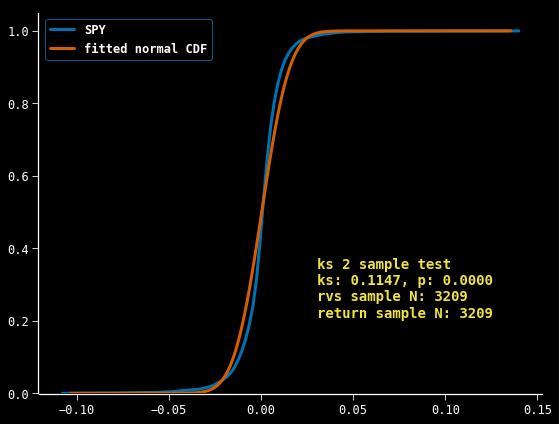This further confirms our assumption that the two series are in fact drawn from seperate distros. Let's look at the same information by year.

In :
def plot_facet_cdf(rs, ex, **kwds):
"""fn: to plot single distro with fitted histograms using FacetGrid

# Args
rs : pd.DataFrame(), returns dataframe
ex : str(), security/column name
"""
g = (rs
.pipe(sns.FacetGrid,
col='year',
col_wrap=2,
size=5,
aspect=1.3)
.map(plot_cdf, ex, **kwds))

CPU times: user 4.53 s, sys: 2.32 s, total: 6.85 s